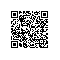# 古典密码算法------替代密码算法

 替代密码算法的原理是使用替代法进行加密，就是将明文中的字符用其它字符替代后形成密文。例如：明文字母a、b、c、d ，用D、E、F、G做对应替换后形成密文。 替代密码包括多种类型，如单表替代密码、多明码替代密码、多字母替代密码、多表替代密码等。下面我们介绍一种典型的单表替代密码，恺撒(caesar)密码，又叫循环移位密码。它的加密方法，就是将明文中的每个字母用此字符在字母表中后面第k个字母替代。它的加密过程可以表示为下面的函数： E(m)=(m+k) mod n 其中：m为明文字母在字母表中的位置数；n为字母表中的字母个数；k为密钥；E(m)为密文字母在字母表中对应的位置数。 例如，对于明文字母H，其在字母表中的位置数为8，设k=4，则按照上式计算出来的密文为L： E(8) = (m+k) mod n = (8+4) mod 26 = 12 = L

1. package cn.hdu.edu.encrypt;
2.
3.
4. /*
5.  * 古典密码算法之  替代算法
6.  *
7.  */
8. public class Replace {
9.
10.     //当加密或者解密成功时返回CRYPT_OK，失败时返回CRYPT_ERROR
11.     private final static int CRYPT_OK =     1;
12.     private final static int CRYPT_ERROR = 0;
13.     //设定一共有26个可用字母  字母a的编码是97
14.     private final static int totalLetter = 26;
15.     private final static int key = 3;
16.
17.     /*
18.      * @param initCode 没有加密前的字符串
19.      * @CRYPT_OK 加密成功
20.      * @CRYPT_ERROR 加密失败
21.      */
22.     public static int encrypt(String initCode)throws Exception{
23.     //用来输出加密后的字符
24.     StringBuilder sb = new StringBuilder();
25.     for(int i = 0; i < initCode.length(); i++ ){
26.             int initCodeVal = initCode.charAt(i ) - 96;
27.             int targetCodeVal = (initCodeVal + key ) % totalLetter;
28.             if(targetCodeVal == 0) targetCodeVal = 26;
29.             char targetCode =  (char)(targetCodeVal +96);
30.             sb.append(targetCode );
31.     }
32.     //以下就是加密后的字符
33.     String targetCode = sb.toString();
34.     System.out.println(targetCode);
35.         return CRYPT_OK;
36.     }
37.
38.     /*
39.      * @param targetCode 加密前的字符串
40.      * @CRYPT_OK 解密成功
41.      * @CRYPT_ERROR 解密失败
42.      */
43.     public static int decrypt(String targetCode)throws Exception{
44.     //用来输出解密后的字符
45.     StringBuilder sb = new StringBuilder();
46.     for(int i = 0; i < targetCode.length(); i++ ){
47.             int targetCodeVal = targetCode.charAt(i ) - 96;
48.
49.             //如果已经来到了字母表的开头   给他还回去
50.             if(targetCodeVal <= key)     targetCodeVal += 26;
51.
52.             int initCodeVal = targetCodeVal - key;
53.             char initCode =  (char)(initCodeVal + 96);
54.             sb.append(initCode );
55.     }
56.     //以下就是解密后的字符
57.     String initCode = sb.toString();
58.     System.out.println(initCode);
59.         return CRYPT_OK;
60.     }
61.
62.
63.
64.
65. }使用钉钉扫一扫加入圈子
+ 订阅